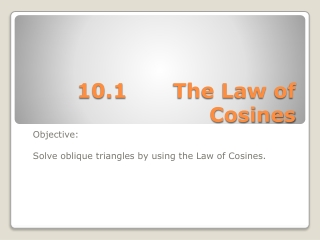DownloadDownload Presentation10.1 The Law of Cosines

# 10.1 The Law of Cosines

Télécharger la présentation## 10.1 The Law of Cosines

- - - - - - - - - - - - - - - - - - - - - - - - - - - E N D - - - - - - - - - - - - - - - - - - - - - - - - - - -
##### Presentation Transcript

1. 10.1 The Law of Cosines Objective: Solve oblique triangles by using the Law of Cosines.

2. The Law of Cosines

3. Label all sides and angles using standard notation. R p t Standard Notation

4. Solve triangle ABC shown below: Ex. #1 Solve a Triangle with SAS Information

5. Find the angles of triangle RST shown below: Ex. #2 Solve a Triangle with SSS Information

6. Find the angles of triangle RST shown below: Ex. #2 Solve a Triangle with SSS Information

7. A ship R leaves port P at a rate of 16 miles per hour. Another ship Q leaves the same port at the same time and travels 12 miles per hour. Both ships are traveling in straight paths. The direction taken by the two ships makes an angle of 55°. How far apart are the ships after 3 hours? In 3 hrs, ship R will have traveled 3(16) = 48 miles, and ship Q will have traveled 3(12) = 36 miles. Ex. #3 The Distance Between Two Vehicles

8. A factory conveyor belt that makes an angle of 17° with the horizontal and rises from point Q on level 1 to point S on level 2 must be replaced. A design change calls for a vertical post RT and dimensions as shown in the diagram below. A new conveyor belt will be needed to go from Q to R to S. What will be the length of the new conveyor belt? 90°−17° = 73° 180°−73° = 107° 73° 107° 73° Ex. #4 Find Angles with the Horizontal

9. A new conveyor belt will be needed to go from Q to R to S. What will be the length of the new conveyor belt? 73° 107° The length of the new conveyor belt will be: 91.8 + 64.8 = 156.6. Ex. #4 Find Angles with the Horizontal